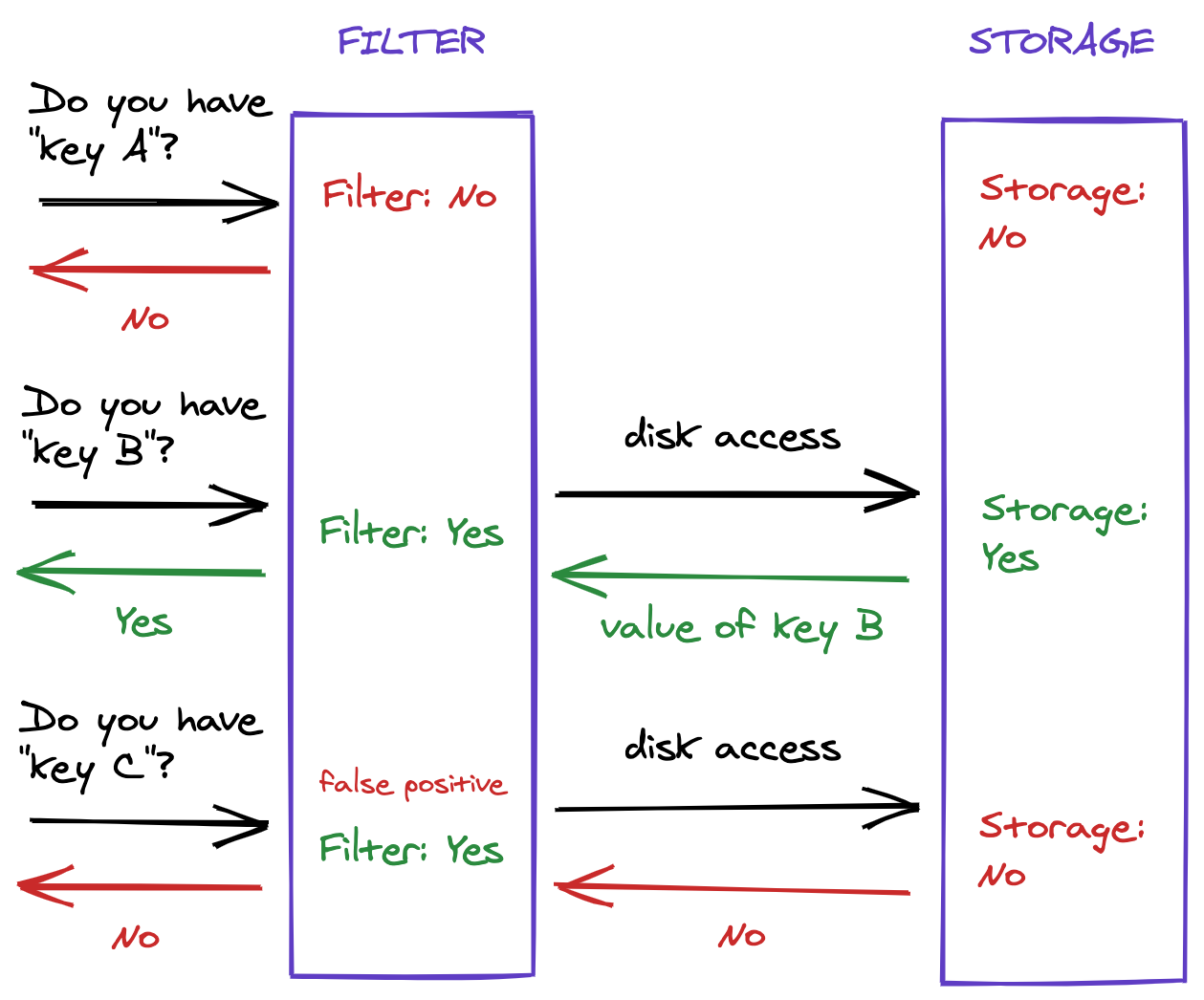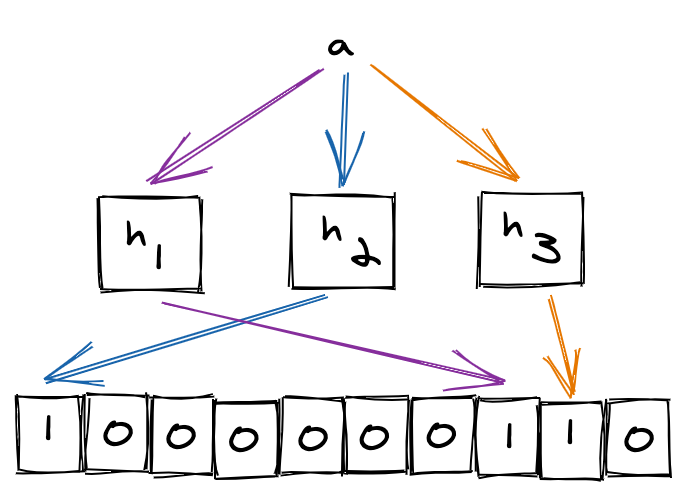I am planning to create a series of blog posts that includes some literature research, implementation of various data structures and our journey of creating a distributed datastore in distrentic.io.

You might be wondering why I start with a blog post explaining the Bloom Filter while I don’t have single clue about how to create a distributed datastore? My answer is simple: “I like the idea behind it”.

Before I get into the details of the Bloom filters, I want to give our backstory that will help you understand why we started building something we’d enjoy during our spare time that will never be production ready.

#### The backstory

My friend Ibrahim and I are always fascinated by complex software and distibuted systems - We’ve been working together more than 5 years (we got old dude) and we were lucky enough to work for the largest e-commerce company in Europe. We battled our way solving many different problems that distributed systems can offer. We both moved to Cambridge, UK and still fighting against distributed world villains.

Let’s explore the mystic land of probabilistic data structures by implementing a Bloom Filter.

## What the hell is a Bloom Filter

You might also want to read Bloom filters debunked.

A Bloom filter is a method for representing a set $A = {a_1, a_2,\ldots, a_n}$ of n elements (also called keys) to support membership queries. It was invented by Burton Bloom in 1970 and was proposed for use in the web context by Marais and Bharat as a mechanism for identifying which pages have associated comments stored within a CommonKnowledge server. 1

It is a space-efficient probabilistic data structure that is used to answer a very simple question: is this element a member of a set?. A Bloom filter does not store the actual elements, it only stores the membership of them.

False positive matches are possible, but false negatives are not – in other words, a query returns either “possibly in set” or “definitely not in set”. 2 Unfortunately, this also means items cannot be removed from the Bloom Filter (Some other element or group of elements may be hashed to the same indices).

Because of its nature of being probabilistic, the Bloom Filter trades space and performance for accuracy. This is much like the CAP theorem, we choose performance over accuracy.

Bloom filters have some interesting use cases. For example, they can be placed on top of a datastore. When a key is queried for its existence and the filter does not have it, we can skip querying the datastore entirely.Figure 1: Example usage of a Bloom filter.

### How does it work

The idea behind Bloom filter is very simple: Allocate an array $v$ of $m$ bits, each bit in the array is initially set to $0$, and then choose $k$ independent hash functions $h_1, h_2, …, h_k$, each with range ${1,…,m}$.

The Bloom filter has two operations just like a standard set:

#### Insertion

When an element $a \in A$ is added to the filter, the bits at positions $h_1(a), h_2(a), …, h_k(a)$ in $v$ are set to $1$. In simpler words, the new element is hashed by $k$ number of functions and modded by $m$, resulting in $k$ indices into the bit array. Each bit at the respective index is set.Figure 2: Adding elements to a Bloom filter ($m = 10$, $k = 3$).

#### Query

To query the membership of an element $b$, we check the bits at indices $h_1(b), h_2(b), …, h_k(b)$ in $v$. If any of them is $0$, then certainly $b$ is not in the set $A$. Otherwise, we assume that $b$ is in the set although it’s possible that some other element or group of elements hashed to the same indices. This is called a false positive. We can target a specific probability of false positives by selecting an optimal value of $m$ and $k$ for up to $n$ insertions.

A Bloom filter eventually reaches a point where all bits are set, which means every query will indicate membership, effectively making the probability of false positives $1$. The problem with this is it requires a priori knowledge of the data set in order to select optimal parameters and avoid “overfilling”. 3

### Finding optimal $k$ and $m$

We can derive optimal $k$ and $m$ based on $n$ and a chosen probability of false positives $P_{FP}$.

$k = -\frac{\ln{P_{FP}}}{\ln{2}} , m = -\frac{n\ln{P_{FP}}}{(\ln2)^2}$

If you want to learn about how the above formulae are derived, you might want to pay a visit here.

## Rust implementation

You can find the full implementation here. Huge thanks to @xfix for fixing hashers initialization with the same seed and @dkales for spotting an issue with the ordering of operations in index calculation.

Finally! It is time to write some rust. I am simultaneously implementing the bloom filter whilst writing this blog post. If you don’t believe me then check the below command:

cargo new --lib plum


Let’s continue with the dependencies. There is only one dependency and we will use it to create $v$.

[dependencies]
bit-vec = "0.6"


We will declare a new struct StandardBloomFilter to encapsulate required fields $k$ (optimal number of hash functions), $m$ (optimal size of the bit array), $v$ (the bit array), hash functions and a marker to tell rust compiler that our struct “owns” a T.

extern crate bit_vec;

use bit_vec::BitVec;
use std::collections::hash_map::{DefaultHasher, RandomState};
use std::hash::{BuildHasher, Hash, Hasher};
use std::marker::PhantomData;

pub struct StandardBloomFilter<T: ?Sized> {
bitmap: BitVec,
optimal_m: u64,
optimal_k: u32,
hashers: [DefaultHasher; 2],
_marker: PhantomData<T>,
}


Careful readers will think that I made a mistake in the declaration of hashers array because of the requirement of $k$ independent hash functions. It was indeed intentional here’s why:

Why two hash functions? Kirsch and Mitzenmacher demonstrated in their paper that using two hash functions $h_1(x)$ and $h_2(x)$ to simulate additional hash functions of the form $g_i(x) = h_1(x) + {i}{h_2(x)}$ can be usefully applied to Bloom filters. This leads to less computation and potentially less need for randomness in practice. 4 This formula may appear similar to the use of pairwise indenpendent hash functions. Unfortunately, there is no formal connection between the two techniques.

I mentioned earlier that the Bloom Filter has two operations like a standard set: insert and query. We will implement those two operations along with constructor-like new method.

impl<T: ?Sized> StandardBloomFilter<T> {
pub fn new(items_count: usize, fp_rate: f64) -> Self {
// ...snip
}
}


new calculates the size of the bitmap ($v$) and optimal_k ($k$) and then instantiates a StandardBloomFilter.

impl<T: ?Sized> StandardBloomFilter<T> {
pub fn new(items_count: usize, fp_rate: f64) -> Self {
let optimal_m = Self::bitmap_size(items_count, fp_rate);
let optimal_k = Self::optimal_k(fp_rate);
let hashers = [
RandomState::new().build_hasher(),
RandomState::new().build_hasher(),
];
StandardBloomFilter {
bitmap: BitVec::from_elem(optimal_m as usize, false),
optimal_m,
optimal_k,
hashers,
_marker: PhantomData,
}
}

// ...snip

fn bitmap_size(items_count: usize, fp_rate: f64) -> usize {
let ln2_2 = core::f64::consts::LN_2 * core::f64::consts::LN_2;
((-1.0f64 * items_count as f64 * fp_rate.ln()) / ln2_2).ceil() as usize
}

fn optimal_k(fp_rate: f64) -> u32 {
((-1.0f64 * fp_rate.ln()) / core::f64::consts::LN_2).ceil() as u32
}

// ...snip
}


Let’s run these calculations on Rust Playground.

bitmap_size: 9585059
optimal_k: 7


This looks really promising! A Bloom Filter that represents a set of $1$ million items with a false-positive rate of $0.01$ requires only $9585059$ bits ($~1.14\mathrm{MB}$) and 7 hash functions.

We managed to construct a Bloom Filter so far and it is time to implement insert and contains methods. Their implementations are dead simple and they share the same code to calculate indexes of the bit array.

impl<T: ?Sized> StandardBloomFilter<T> {
// ...snip

pub fn insert(&mut self, item: &T)
where
T: Hash,
{
let (h1, h2) = self.hash_kernel(item);

for k_i in 0..self.optimal_k {
let index = self.get_index(h1, h2, k_i as u64);

self.bitmap.set(index, true);
}
}

pub fn contains(&mut self, item: &T) -> bool
where
T: Hash,
{
let (h1, h2) = self.hash_kernel(item);

for k_i in 0..self.optimal_k {
let index = self.get_index(h1, h2, k_i as u64);

if !self.bitmap.get(index).unwrap() {
return false;
}
}

true
}
}


The above methods depend on two other methods that we haven’t implemented yet: hash_kernel and get_index. hash_kernel is going to be the one where the actual “hashing” happens. It will return the hash values of $h_1(x)$ and $h_2(x)$.

impl<T: ?Sized> StandardBloomFilter<T> {
// ...snip

fn hash_kernel(&self, item: &T) -> (u64, u64)
where
T: Hash,
{
let hasher1 = &mut self.hashers.clone();
let hasher2 = &mut self.hashers.clone();

item.hash(hasher1);
item.hash(hasher2);

let hash1 = hasher1.finish();
let hash2 = hasher2.finish();

(hash1, hash2)
}
}


We could’ve used $128$ bit MurmurHash3 and returned upper $64$ bit as hash1 and the lower as hash2 but to keep this implementation even simpler (this is how Google Guava Bloom Filter implementation currently works) and not to rely on any other additional dependencies I decided to continue with DefaultHasher - see SipHash

Now, it is time to make the final touch. We are going to implement get_index by using $g_i(x) = h_1(x) + {i}{h_2(x)}$ to simulate more than two hash functions.

impl<T: ?Sized> StandardBloomFilter<T> {
// ...snip

fn get_index(&self, h1: u64, h2: u64, k_i: u64) -> usize {
}

// ...snip


### We are finally thereWe’ve just finished implementing a fast variant of a standard Bloom Filter but there is still one thing missing - We didn’t write any tests.

Let’s add two simple test cases and validate our implementation.

#[cfg(test)]
mod tests {
use super::*;

#[test]
fn insert() {
let mut bloom = StandardBloomFilter::new(100, 0.01);
bloom.insert("item");
assert!(bloom.contains("item"));
}

#[test]
fn check_and_insert() {
let mut bloom = StandardBloomFilter::new(100, 0.01);
assert!(!bloom.contains("item_1"));
assert!(!bloom.contains("item_2"));
bloom.insert("item_1");
assert!(bloom.contains("item_1"));
}
}

❯ cargo test
Compiling plum v0.1.2 (/Users/onat.mercan/dev/distrentic/plum)
Finished test [unoptimized + debuginfo] target(s) in 0.99s
Running target/debug/deps/plum-6fc161db530d5b36

running 2 tests
test tests::insert ... ok
test tests::check_and_insert ... ok

test result: ok. 2 passed; 0 failed; 0 ignored; 0 measured; 0 filtered out


I hope you’ve enjoyed reading this post as much as I enjoyed writing it!

If you find anything wrong with the code, you can file an issue or, even better, submit a pull request.

Discuss it on HN